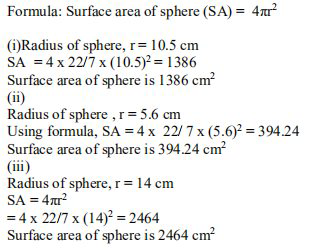# NCERT Solutions Class 9 Mathematics Solutions for Surface Areas and Volumes - Exercise 13.4 in Chapter 13 - Surface Areas and Volumes

Question 1 Surface Areas and Volumes - Exercise 13.4

Find the surface area of a sphere of radius:

(i) 10.5cm (ii) 5.6 cm (iii) 14cm

(Assume π=22/7)Video transcript
"hello students welcome to lido q a video session i am saf your maths tutor and question for today is find the surface area of the sphere of radius first 10.5 centimeter second 5.6 centimeter third 14 centimeter assume pi is equal to 22 by 7 here general formula for the surface area of the sphere is 4 pi r square now in first one the radius is given the 10.5 centimeter so r is equal to ten point five centimeter so surface area is four pi r square 4 into 22 by 7 pi value is 22 by 7 and r is 10.5 and square so solving this you will get one three eight six one three eight six centimeter square in the second one you have radius as 5.6 centimeter so r is equal to 5.6 centimeter surface area is 4 pi r square substitute the values you will get 4 into 22 by 7 into 5.6 square so this is 394.24 centimeter square in third one radius is given that is 14 centimeter surface area is again four pi r square substitute the values and you will get 4 into 22 by 7 into 14 square and this is 2464 centimeter square this is our final answer for all the three sub questions if you have any queries you can drop it in our comment section and subscribe to lido for more such q a thank you for watching "
Related Questions

Lido

Courses

Teachers

Book a Demo with us

Syllabus

Maths
CBSE
Maths
ICSE
Science
CBSE

Science
ICSE
English
CBSE
English
ICSE
Coding

Terms & Policies

Selina Question Bank

Maths
Physics
Biology

Allied Question Bank

Chemistry
Connect with us on social media!